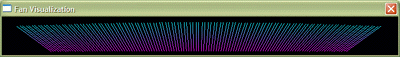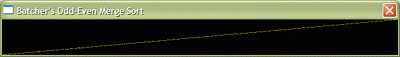| Home | Sign up! | Projects | Seminars | Research Notes | Today's Lecture | About |
 Member ID: 15

## Parul Yadav

Email
parulyadav7@yahoo.co.in

Profile:

Alumnus of AIT.

Alumnus of Coastal Carolina University.

Prof. Ashay Dharwadker's Courses (3):

 Course Semester Grade Add New Course Probability & Statistics Spring 2003 View Update Course Algorithm Design - I Fall 2002 View Update Course Algorithm Design - II Spring 2004 View Update Course

### Projects (1)

 Project ID: 48Course: Algorthim Design - IITopic: Sorting Algorithms VisualizationUpdate Project Description: In this project we have tried to implement the sorting techniques using different visualizations. I have made a graphical visualization in the shape of a fan. It consists of lines which are inclined at different angles and finally, after each sorting method is implemented, they get inclined in a sorted manner. Over here the visualization shown is that of Merge Sort.Team Manifesto The first (and probably the most important) thing that any computer programmer learns are the various algorithms for SORTING data. They are absolutely critical for a complete understanding of the whole computer science field itself. But it can get quite confusing at times. Especially, when one wants to understand how each one of them works? Or, How each one of them is different from the other? We think we have an answer to that. Suppose you wish to introduce somebody to the game of FOOTBALL. A verbal discription would not be very helpful. The best solution would be to show a visual of the football game to that person. Same is the case with Sorting Methods. Through this project, we give a visual discription of the various Sorting Algorithms, with the aim of making learning easy and fun. But the solution also gives rise to a more complex problem. How do we represent the sorting algorithms visually. The solution is to create unique visualization schemes to represent the status of data elements at various instances of time. This method of representation gives rise to a more complex field in mathematics known as Dynamical Systems. After the main idea of the project was concieved, the elements of the project were distributed to the various team members. Team Members: • Karan Sahni (Algorithms - Coding Sorting Algorithms into C++ Programs) • Parul Yadav (Visualizations - Creating Visualization Schemes in OpenGL) • Devina Sibal (Visualizations - Creating Visualization Schemes in OpenGL) • Prakash (Win32 Integration - Integrating all components in Visual C++ Environment)

### Research Notes (2)

Research Note ID: 52
Course: Algorithm Design - II
Topic: Hashing and its applications
Description: Hashing is the transformation of a string of characters into a usually shorter fixed-length value or key that represents the original string. Hashing is used to index and retrieve items in a database because it is faster to find the item using the shorter hashed key than to find it using the original value. It is also used in many encryption algorithms.

 A simple example of this will be searching of names in a database consisting of following names: Richa, Poorti,Kavita, Jitin, Pankaj, Preeti Each of these names would be the key in the database for that person's data. A database search mechanism would first have to start looking character-by-character across the name for matches until it found the match (or ruled the other entries out). But if each of the names were hashed, it might be possible (depending on the number of names in the database) to generate a unique four-digit key for each name. For example:  2345 richa 7809 pankaj,jitin 5309 poorti 1904 kavita A search for any name would first consist of computing the hash value (using the same hash function used to store the item) and then comparing for a match using that value. It would, in general, be much faster to find a match across four digits, each having only 10 possibilities, than across an unpredictable value length where each character had 26 possibilities. A hash function H is a transformation that takes an input m and returns a fixed-size string, which is called the hash value h (that is, h = H(m)). The hashing algorithm is called the hash function The hash function is used to index the original value or key and then used later each time the data associated with the value or key is to be retrieved. A good hash function also should not produce the same hash value from two different inputs. If it does, this is known as a collision.
 Here are some relatively simple hash functions that have been used:  The division-remainder method: The size of the number of items in the table is estimated. That number is then used as a divisor into each original value or key to extract a quotient and a remainder. The remainder is the hashed value. (Since this method is liable to produce a number of collisions, any search mechanism would have to be able to recognize a collision and offer an alternate search mechanism.)  Folding: This method divides the original value (digits in this case) into several parts, adds the parts together, and then uses the last four digits (or some other arbitrary number of digits that will work ) as the hashed value or key.  Radix transformation: Where the value or key is digital, the number base (or radix) can be changed resulting in a different sequence of digits. (For example, a decimal numbered key could be transformed into a hexadecimal numbered key.) High-order digits could be discarded to fit a hash value of uniform length.  Digit rearrangement: This is simply taking part of the original value or key such as digits in positions 3 through 6, reversing their order, and then using that sequence of digits as the hash value or key.
 Applications: Hashing has got a lot of applications in the real world: One is searching in database which has been discussed earlier. Hashing is used in cryptography. The basic requirements for a cryptographic hash function are as follows: The input can be of any length. The output has a fixed length. H(x) is relatively easy to compute for any given x. H(x) is one-way. H(x) is collision-free. A hash function H is said to be one-way if it is hard to invert, where "hard to invert'' means that given a hash value h, it is computationally infeasible to find some input x such that H(x) = h. If, given a message x, it is computationally infeasible to find a message y not equal to x such that H(x) = H(y), then H is said to be a weakly collision-free hash function. A strongly collision-free hash function H is one for which it is computationally infeasible to find any two messages x and y such that H(x) = H(y). The hash value represents concisely the longer message or document from which it was computed, this value is called the message digest. The main role of a cryptographic hash function is in the provision of message integrity checks and digital signatures. Now what actually happens in this case is basically the digital signature or integrity check of some document is computed by applying the cryptographic processing to the documents’hash value,which is small compared to the documents itself. Hence in this way a digest can be revealed without actually showing the document from which it is derived.Damgård and Merkle greatly influenced cryptographic hash function design by defining a hash function in terms of what is called a compression function. A compression function takes a fixed-length input and returns a shorter, fixed-length output. Given a compression function, a hash function can be defined by repeated applications of the compression function until the entire message has been processed. In this process, a message of arbitrary length is broken into blocks whose length depends on the compression function, and ``padded'' (for security reasons) so the size of the message is a multiple of the block size. The blocks are then processed sequentially, taking as input the result of the hash so far and the current message block, with the final output being the hash value for the message.

Reference: http://whatis.database.search.co

 Research Note ID: 56Course: Algorithm Design - IITopic: Uses of Hashing in BiologyUpdate Research Note Description: One of the major functions of hashing comes in Structural Biology where it is used to find the conformation of drug molecules (ligands) when they bind to protein surfaces. It is known that the geometric shape of the two molecules at the binding site should be complementary in order for the binding process to occur. It should be taken into account that both the molecules should be complementary to each other in order to fit into each other and for the binding process to occur. There should be the match between ligand and the protein in the docked conformation. Also it may be the case that molecules may change their shape upon binding thus causing the so called induced fit .therefore the structure of the molecule when it is not bound may be different from the bound conformation adding more complexity to the problem. The algorithm requires a surface representation for both the ligand and the receptor. The surfaces are represented by critical points, accurately and sparsely placed, at key locations on the molecular surface. In the algorithm, there are two phases, as before: In the preprocessing phase, the ligand molecules are preprocessed, and their geometrical information is encoded in the hash table. Then, in the recognition phase, the features of the receptor are used to obtain an index to hash table, and the corresponding entries in the hash table receive a vote. At the end of the process, the ligand molecules that receive the highest number of votes can be selected for a more careful analysis. References : Efficient Computational Algorithms for Docking, and for Generating and Matching a Library of Functional Epitopes I. Rigid and Flexible Hinge-Bending Docking Algorithms. Ruth Nussinov, and Haim J. Wolfson. Combinatorial Chemistry & High Throughput Screening, 2, 277-287, (1999).Geometric Hashing: An overview. Haim J. Wolfson, Isidore Rigoutsos. IEEE Computational Science and Engineering, 1997, pp. 10-21

Last updated on Thursday, 10th November 2005, 07:44:07 PM.